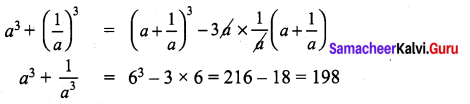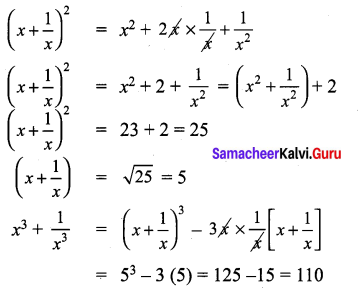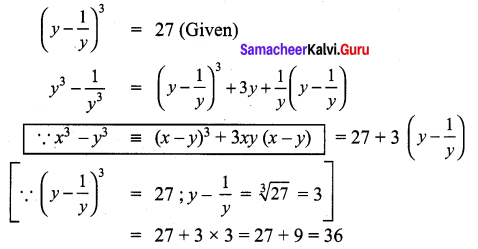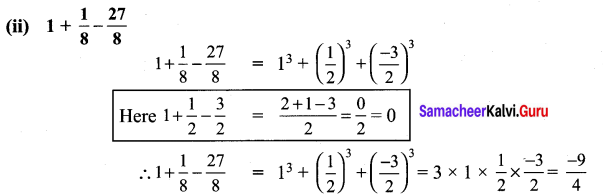# Samacheer Kalvi 9th Maths Solutions Chapter 3 Algebra Ex 3.4

## Tamilnadu Samacheer Kalvi 9th Maths Solutions Chapter 3 Algebra Ex 3.4

9th Maths Algebra Exercise 3.4 Question 1.
Expand the following :
(i) (2x + 3y + 4z)2
(ii) (-p + 2q + 3r)2
(iii) (2p + 3)(2p – 4)(2p – 5)
(iv) (3a + 1)(3a – 2)(3a + 4)
Solution:
(i) (2x + 3y + 4z)2
(a + b + c)2 ≡ a2 + b2 + c2 + 2ab + 2bc + 2ca
∴ (2x + 3y + 4z)2 = (2x)2 + (3y)2 + (4z)2 + 2 (2x) (3y) + 2 (3y) (4z) + 2 (4z) (2x)
= 4x2 + 9y2 + 16z2 + 12xy + 24yz + 16zx

(ii) (-p + 2q + 3r)2
(a + b + c)2 ≡ a2 + b2 + c2 + 2ab + 2bc + 2ca
(-p + 2q + 3r)2 = (-p)2 + (2q)2 + (3r)2 + 2(-p) (2q) + 2(2q) (3r) + 2 (-p) (3r)
= p2 + 4q2 + 9r2 – 4pq +12qr – 6rp

(iii) (2p + 3)(2p – 4)(2p – 5)
(x + a) (x + b) (x + c) ≡ x3 + (a + b + c) x2 + (ab + be + ca) x + abc
(2p + 3)(2p – 4)(2p – 5) = (2p)3 + (3 – 4 – 5) (2p)2 + [(3 × – 4)2 + (-4 × -5) + (-5 × 3)] 2p + 3 × – 4 × – 5
= 8p3 + (-6) (4p2) + [-12 + 20 + (-15)] 2p + 60
= 8p3 – 24p2 + (-7)2p + 60
(2p + 3)(2p – 4)(2p – 5) = 8p3 – 24p2 – 14p + 60

(iv) (3a + 1)(3a – 2)(3a + 4)
(x + a) (x + b) (x + c) ≡ x3 + (a + b + c) x2 + (ab + bc + ca) x + abc
(3a + 1)(3a – 2)(3a + 4) = (3a)3 + (1 – 2 + 4) (3a)2 + [1 × (- 2) + (-2 × 4) + 4 × 1] (3a) + 1 × -2 × 4
= 27a3 + 3 (9a2) + (-2 – 8 + 4)3a – 8
= 27a3 + 27a2 – 8a – 8

9th Maths Exercise 3.4 Question 2.
Using algebraic identity, find the coefficients of x2, x and constant term without actual expansion.
(i) (x + 5)(x + 6)(x + 7)
(ii) (2x + 3)(2x – 5)(2x – 6)
Solution:
(i) (x + 5)(x + 6)(x + 7)
(x + a) (x + b) (x + c) ≡ x3 + (a + b + c) x2 + (ab + bc + ca) x + abc
Co-efficient of x2 = a + b + c = 5 + 6 + 7 = 18
Co-efficient of x2 = ab + bc + ca = (5 × 6) + (6 × 7) + (7 × 5)
= 30 + 42 + 35 = 107
Constant term = abc = 5 × 6 × 7
Co-efficient of constant term = 210

(ii) (2x + 3)(2x – 5)(2x — 6)
∴ Co-efficient of x2 = 4 (a + b + c) = 4 (3 + (-5) + (-6))
= 4 × (-8) = -32
Co-efficient of x = 2 (ab + bc + ca)
= 2 [3 × (-5) + (-5) (-6) + (-6) (3)]
= 2[-15 + 30- 18] = 2 × (-3) = -6
Constant term = abc = 3 × (-5) × (-6) = 90

Class 9 Maths Exercise 3.4 Solutions Question 3.
If (x + a)(x + b)(x + c) = x3 + 14x2 + 59x + 70, find the value of
(i) a + b + c
(ii) $$\frac{1}{a}+\frac{1}{b}+\frac{1}{c}$$
(iii) a2 + b2 + c2
(iv) $$\frac{a}{b c}+\frac{b}{a c}+\frac{c}{a b}$$
Solution:
(x + a)(x + b)(x + c) = x3 + 14x2 + 59x + 70 …………….. (1)
(x + a) (x + b) (x + c) ≡ x3 + (a + b + c) x2 + (ab + bc + ca) x + abc …………. (2)
(i) Comparing (1) & (2)
We get, a + b + c = 14
(ii) $$\frac{1}{a}+\frac{1}{b}+\frac{1}{c}=\frac{b c+a c+a b}{a b c}=\frac{59}{70}$$
(iii) (a + b + c)2 = a2 + b2 + c2 + 2ab + 2bc + 2ca
(a + b + c)2 = a2 + b2 + c2 + 2(ab + bc + ca)
= 142 – 2 (59) = 196 – 118 = 78
(iv) $$\frac{a}{b c}+\frac{b}{a c}+\frac{c}{a b}=\frac{a^{2}+b^{2}+c^{2}}{a b c}=\frac{78}{70}=\frac{39}{35}$$

9th Class Maths Exercise 3.4 Solution Question 4.
Expand
(i) (3a – 4b)3
(ii) (x + $$\frac{1}{y}$$)3
Solution:
(i) (3a – 4b)3 We know that
(x – y)3 = x3 – 3x2y + 3xy2 – y3
(3a-4b)3 = (3a)3 – 3 (3a)2 (4b) + 3 (3a) (4b)2 – (4b)3
= 27a3 – 108a2b + 144 ab2 – 64b3

(ii) (x + $$\frac{1}{y}$$)3
(x + y)3 ≡ x3 + 3x2y + 3xy2 + y3
$$\left(x+\frac{1}{y}\right)^{3}=x^{3}+\frac{3 x^{2}}{y}+\frac{3 x}{y^{2}}+\frac{1}{y^{3}}$$

Exercise 3.4 Class 9 Maths Solution Question 5.
Evaluate the following by using identities:
(i) 983
(ii) 10013
Solution:
(i) 982 = (100 – 2)3
(a – b)3 ≡ a3 – 3a2b + 3ab2 – b3
983 = (100 – 2)3 = 1003 – 3 × 1002 × + 3 × 100 × 22 – 23
= 1000000 – 3 × 10000 × 2 + 300 × 4 – 8
= 1000000 – 60000 + 1200 – 8 = 1001200 – 60008 = 941192

(ii) 10013 = (1000 + 1)3
(a + b)3 ≡ a3 + 3a2b + 3ab2 + b3
(1000 + 1)3 = 10003 + 3(10002) × 1 + 3 × 1000 × 12 + 13
= 1000,000,000 + 3,000,000 + 3000 + 1 = 1,003,003,001

9th Standard Maths Exercise 3.4 Question 6.
If (x + y + z) = 9 and (xy + yz + zx) = 26 then find the value of x2 + y2 + z2.
Solution:
(x + y + z) = 9 and(xy + yz + zx) = 26
x2 + y2 + z2 = (x + y + z)2 – 2 (xy + yz + zx)
= 92 – 2 × 26 = 81 – 52 = 29

9th Class Math Exercise 3.4 Solution Question 7.
Find 27a3 + 64b3, if 3a + 4b = 10 and ab = 2.
Solution:
3a + 4b = 10, ab = 2
(3a + 4b)3 = (3a)3 + 3 (3a)2 (4b) + 3 (3a) (4b)2 + (4b)3
(27a3 + 64b3) = (3a + 4b)3 – 3 (3a) (4b) (3a + 4b)
∵ x3 + y3 = (x + y)3 – 3xy – (x + y)
= 103 – 36 ab (10)= 1000 – 36 × 2 × 10
= 1000 – 720 = 280

Maths Class 9 Chapter 3 Exercise 3.4 Question 8.
Find x3 – y3, if x – y = 5 and xy = 14.
Solution:
x – y = 5, xy = 14
x3 – y3 = (x – y)3 + 3xy (x – y) = 53 + 3 × 14 × 5
= 125 + 210 = 335

Maths Exercise 3.4 Class 9 Question 9.
If a + $$\frac{1}{a}$$ = 6, then find the value of a3 + $$\frac{1}{a^{3}}$$
Solution:
a3 + b3 = (a + b)3 – 3ab (a + b)9th Class Math Ex 3.4 Solution Question 10.
If x2 + $$\frac{1}{x^{2}}$$ = 23, then find the value of x + $$\frac{1}{x}$$ and x3 + $$\frac{1}{x^{3}}$$
Solution:9th Class Math, Exercise 3.4 Solution Question 11.
If (y – $$\frac{1}{y}$$)3 = 27, then find the value of y3 – $$\frac{1}{y^{3}}$$
Solution:9th Class Math Chapter 3 Exercise 3.4 Question 12.
Simplify : (i) (2a + 36 + 4c)(4a2 + 9b2 + 16c2 – 6ab – 12bc – 12bc – 8ca)
(ii) (x – 2y + 3z) (x2 + 4y2 + 9z2 + 2xy + 6yz – 3xz)
Solution:
(i) (2a + 36 + 4c)(4a2 + 9b2 + 16c2 – 6ab – 12bc – 12bc – 8ca)
We know that
(a + b + c) (a2 + b2 + c2 – ab – bc – ca) = a3 + b3 + c3 × 3 abc
∴ (2a + 36 + 4c) (4a2 + 9b2 + 16 c2 – 6 ab – 12 bc – 8 ca)
= (2a)3 + (3b)3 + (4c)3 – 3 × 2a × 36 × 4c
= 8a3 + 27b3 + 64c3 – 72 abc

(ii) (x – 2,y + 3z) (x2 + 4y2 + 9z2 + 2xy + 6yz – 3xz)
(a + b + c) (a2 + b2 + c2 – ab – bc – ca) = a3 + b3 + c3 – 3abc .
∴ (x – 2y + 3z) (x2 + 4y2 + 9z2 + 2xy + 6yz – 3xz)
= x3 + (-2y)3 + (3z)3 – 3 × x × (-2y) (3z)
= x3 – 8y3 + 27z3 + 18 xyz

9 Class Math 3.4 Solution Question 13.
By using identity evaluate the following:
(i) 73 – 103 + 33
(ii) 1 + $$\frac{1}{8}-\frac{27}{8}$$
Solution:
(i) 73 – 103 + 33
(a + b + c) (a2 + b2 + c2 – ab – bc – ca) = a3 + b3 + c3 – 3 abc
If a + b + c = 0, then a3 + b3 + c3 = 3 abc
∴ 7 – 10 + 3 = 0
⇒ 73 – 103 + 33 = 3 × 7 × – 10 × 3
= 9 × -70 = -630Ex 3.4 Class 9 Maths Solutions Question 14.
If 2x – 3y – 4z = 0, then find 8x3 – 27y3 – 64z3.
Solution:
If 2x – 3y – 4z = 0 then 8x3 – 27y3 – 64z3 = ?
If x + y + z = 0 then x3 + y3 + z3 = 3xyz
8x3 – 21 y3 – 64z3 = (2x)3 + (-3y)3 + (-4z)3
= 3 × 2x × -3y × – 4z = 72 xyz